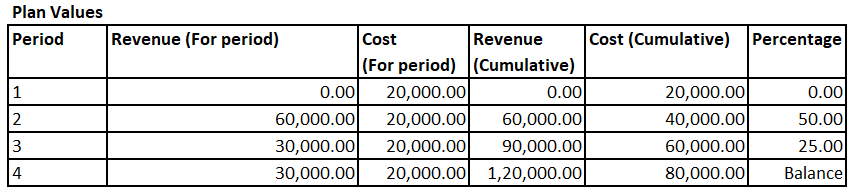Search
•admin

# Result Analysis – Part II – (01) Revenue-Based Method with Profit Realization

## Result Analysis

In the previous post we had an overview of how we will be going about our understanding of of Result Analysis in SAP.

Here, in this article, we will try to conceptually understand how the Valuation Method “01 – Revenue-Based Method with Profit Realization” works. This article will deep dive into the calculations performed by SAP when we use this Valuation method.

## (01) Revenue-Based Method with Profit Realization

The calculations used in this method are based on the actual revenue booked for Project. It calculate proportionate cost based on plan cost/plan revenue in order to calculate Cost of Sales. System then calculates if the Cost of Sales is different than the actual cost, if yes, posts difference to adjust the project cost, this helps achieve the planned profitability throughout the lifespan. But at the end, when we perform Technical Completion (TECO) and Closure of project, system reverses the calculated cost so as to have actual revenue and actual cost appear in the financials.

In order to understand this Method properly we will use an example which will give us better view of calculations performed by system.

## Example

Assumptions: We are considering following values for the example:

### Overall Plan ValuesBefore we go ahead, it is important to understand that SAP uses following formula to calculate Cost of Sales:

Cost of Sales = (Planned Cost X Actual Revenue) / Planned Revenue

### 1st Month

For the first month, we have an actual cost of 20000 but no revenue.When we perform Result Analysis, system calculates cost of sales using following formula

Cost of Sales = (Planned Cost X Actual Revenue) / Planned Revenue

But since we do not have any revenue (both actual and planned) for the period, system calculates zero as Cost of Sales. Hence, it will reverse the expense booked and post an accounting entry to this effect when we perform project settlement.

### 2nd Month

For the second month, we have an actual cost of 25000 which accumulates to 45000 till date and a revenue of 75000 is booked.When we perform Result Analysis, system calculates cost of sales using following formula

Cost of Sales = (Planned Cost X Actual Revenue) / Planned Revenue

In this month we have a cumulative planned cost of 45000 where as Plan Revenue of 60000 and actual Revenue of 75000. Hence the system calculates Cost of Sales as below:

(80000 X 75000) / 120000 which comes to 50000.

Now this value of 50000 is higher than the actual cumulative cost of 45000 booked by us. Hence, system works as below:

1. Reversal of transaction posted in previous period

2. Posting of a new document to book differential expense of 5000 to increase the total cost to 50000

Now due to this increase in cost, we are able to draw the profit of 33.33% which instead would have been different than this.

### 3rd Month

For the third month, we have an actual cost of 20000 which accumulates to 65000 till date and a cumulative revenue of 90000 is booked.When we perform Result Analysis, system calculates cost of sales using following formula

Cost of Sales = (Planned Cost X Actual Revenue) / Planned Revenue

In this month we have a cumulative planned cost of 65000 where as Plan Revenue of 60000 and actual Revenue of 75000. Hence the system calculates Cost of Sales as below:

(80000 X 90000) X 60000 which comes to 60000.

Now this value of 60000 is lower than the actual cumulative cost of 65000 booked by us. Hence, system works as below:

1. Reversal of transaction posted in previous period

2. Posting of a new document to book differential expense of 5000 to reduce the total cost to 60000

Now due to this increase in cost, we are able to draw the profit of 33.33% which instead would have been different than this.

### 4th MonthNow we have reached the last period of the project. During this period we consider that the Project is marked as TECO and Closed. After the change of status, when we perform Result Analysis and do Project settlement, system reverses previous period entries and does not calculate Cost of Sales any further. This helps us to have the actual cost and actual revenue in our financials to get actual profit percentage.

## Conclusion

The above example helps us to understand how calculations are performed by system when we use this Result Analysis Method for Percentage of Completion in Project System. This example also helps us conceptually get acquainted with the way calculates are performed in the system with their logics.

SAP Help